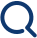# How to Hedge the Impermanent loss in Defi farming

Investment Chapter Author: Tao Huang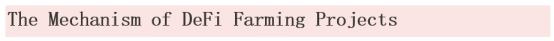In AMMs DEX protocols, users can deposit specific assets into the protocol pools to help provide liquidity for the protocols. We usually call them Liquidity Providers (LPs). When any trader executes trading orders in the protocol, LPs have to passively act as the counterparty and bear the potential losses which we called impermanent loss.

What the LPs do is very similar to the market makers in traditional centralized exchanges. For the P/L of LPs token changes nonlinearly to the price change of the underlying, this market-making model is analogous to the option market.

Here is an example.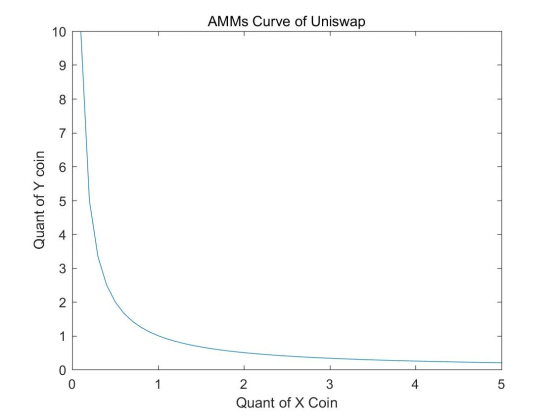It is the basic idea of Uniswap. The quantities of the tokens which the LPs deposit will change according to the traders' behaviors. The core formula is listed below

X*Y=K

X/Y=P

X denotes the quantity of token X. Y is the quantity of token Y. K is a constant, which is the square of the so-called liquidity in Uniswap team's paper. P is the relative price of Y to X.

It is well-known that when LPs have completed the depositing, K would be a constant irrespective of the fee rebate. As long as one of the three variables, ie., X, Y, and P, is assigned with a value, the other two can easily be calculated. Then we can calculate the impermanent loss in this model.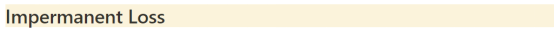The concept of the impermanent loss is proposed based on the NAV of holders. By neglecting the fee rebate, the net value of the LP token will always be less than the value of the token pairs deposited initially.

In the case that NAV or Delta curve is nonlinear, the impermanent loss has to be hedged through the dynamic position of the underlying assets, which means we have to rebalance the hedge position dynamically in response to the moving of the underlying price. Every time when we make the rebalance, it will take friction costs. So, the more frequently we make the hedge, the more friction costs we have to bear. It makes that when we consider the hedge program of impermanent loss, we have to identify the sensibility of impermanent loss to the moving of the underlying price.

At the very beginning, our intuitive thought is that the impermanent loss is really big when liquidity is being provided in AMMs so that we have to take a large risk. Meanwhile, any hedging mechanism would take a large cost which may lead to the overall loss of the portfolio. However, the impermanent loss is absolutely in control. We can take the ETH/USDT pair as an example.

Given that when the ETH price was 2,000 USDT, Alice deposited 10,000 USDT and 5 ETH into the liquidity pool.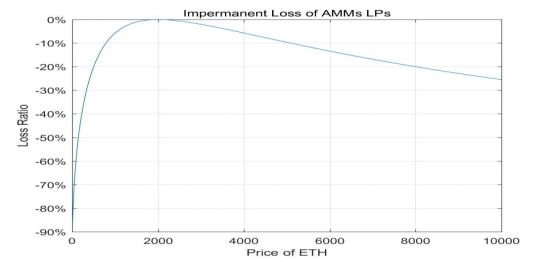According to the figure above, the impermanent loss would be less than 1% when the ETH price is in the range of 1,510 and 2,650 USDT. it means that the potential loss would be less than 1% for ETH price fluctuates between -24.5% and 32.5%. This large range implies that the sensitivity of impermanent loss to the price change is very weak.

Therefore, we conclude that hedging the impermanent loss can be not so costly. Then, we can go to the exact hedge mechanism itself. Considering that the premium fee of the option is very high in the crypto world. we decide to hedge through perpetuals.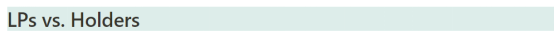Firstly, we have to say that, hedge is not always a good idea because it is costly. As usual, all of the trading strategies should be based on users' basic estimation of the market.

In order to have a deeper understanding of this, we compare the LPs' NAV performance to the holders' firstly. Again, we assume the initial ETH price is 2,000 USDT, and the initial principle is 10,000 USDT and 5 ETH.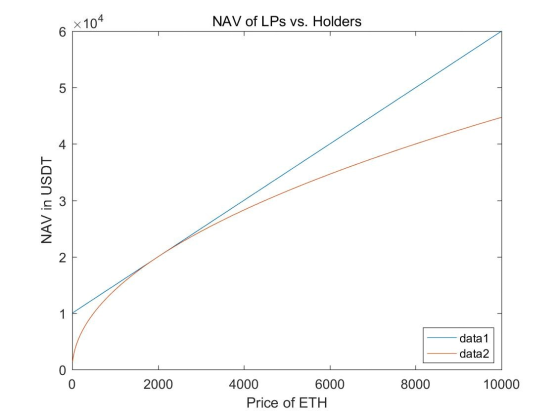In the above figure, data1 denotes the NAV of holders while data 2 is the NAV of LPs. In this figure, we assume no fee rebate and farming yield to simplify the model. We can see that the NAV of LPs would be always less than NAV of holders. But when we take into account fee rebate and farming yield, it would be different.

Now we assume the investment horizon is 3 months, the annual fee rebate is 10%, the annual farming yield is 20%. Let us go back to the figure again.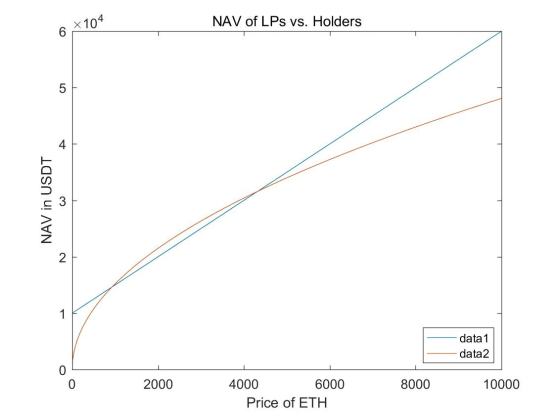When we take into account 30% annual yield, we can see that the LPs will have an advantage over holders when the ETH price is in the range of [930, 4,310] which means [-53.5%, 115.5%]. It is a very wide range, too! Moreover, if we can sustain an annual yield of over 30%, the advantage can be more obvious.

Now we can conclude that holding LP tokens without a hedge is also a very good investment strategy itself because that LP tokens are a type of asset with yields which are very likely to beat the impermanent loss. However, in the situation of large underlying price movement, holding LP tokens will incur terrible losses for the impermanent loss grows exponentially.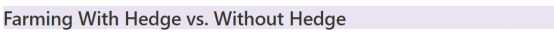The prices of crypto assets are extremely volatile in most of the time. Generally, we cannot be convinced that the price of ETH will not go below 930 or above 4,310 in 3 months. In this case we have to hedge the impermanent loss to eliminate risk.

Firstly, we use a simple model to give a basic idea for the difference of with or without a hedge. We assume that the hedge cost would be 5% annually. Then we go back to the figure again.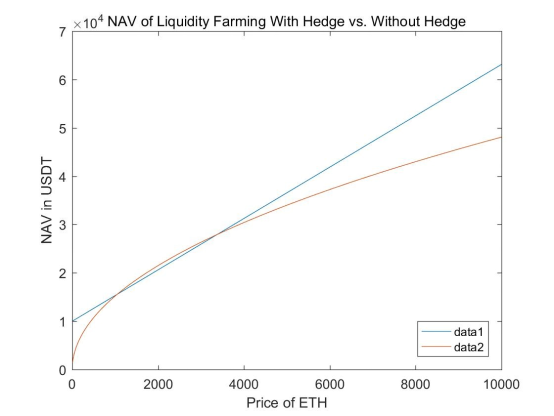We can see that the NAV curve for farming with a hedge is very similar to that of holders. It is because when we perfectly hedge the impermanent loss, the thing will become holders with profits.

We can also see that in the range of [1,060, 3,370] which means [-47%, 68.5%], the NAV of unhedged farming will be higher than that of hedged farming.

It seems to be very likely that farming without a hedge will still beat farming with a hedge. However, we have to notice when the price is in the range of [1,060, 3,370], the NAV of without a hedge is only slightly higher than with a hedge. On the other hand, when the price is far away from this range, the NAV without a hedge will be much lower than with a hedge. This is the core value of a hedge.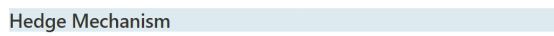As aforementioned, holding LP tokens is very similar to market making of options. Considering this, we choose to hedge the impermanent loss risk by a similar mechanism in option market which is called delta hedge.

Since we know that the LP token's NAV will change nonlinearly with the change of the underlying price, we can easily calculate the delta of LP tokens for multiple underlying prices. Intuitively, we find that the delta of LP tokens will be always 0.5 because LP always hold 50% position of the underlying. According to that, we can build up a grid in each point of which we rebalance the USD delta of the portfolio to that of holder's.

For the impermanent loss, we give the equation of below:

Impermanent Loss = LPs NAV/Holder's NAV-1

For the hedging position, we give the equation of below:

Hedging Position = df(price)/dprice

f(price) = Holder's NAV - LPs NAV

Our basic idea is to open or close the hedge position whenever the impermanent loss is hitting a threshold.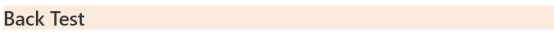Again, we take ETH/USDT pair as an example. We take the Binance Perpetual as the hedge tool. Our hypotheses is listed below:

1. The principle is 1,000,000 USDT.
2. The slippage is 0.5 USDT.
3. The commission rate is 0.017%.
4. The annual farming yield is 20%.
5. The annual rebate rate is 10%.
6. The grid we use is 1%.

Please see the back test result below. The first back test time horizon is from 01.2020 to 07.2021.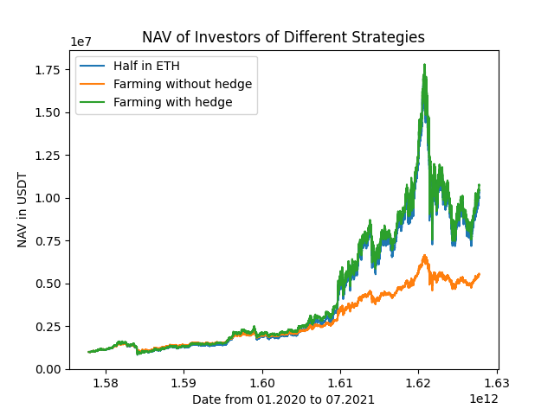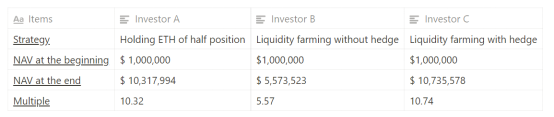During this time horizon, the ETH price grew from \$128.87 to \$2,530.49, which means a 19.63 times increase. This leads to so large an impermanent loss that Investor B's portfolio performance is relatively bad. But with our hedge strategy, Investor C's performance is the best.

The time horizon of our second back test is from 01.2020 to 10.2020, in which the volatility is relatively low.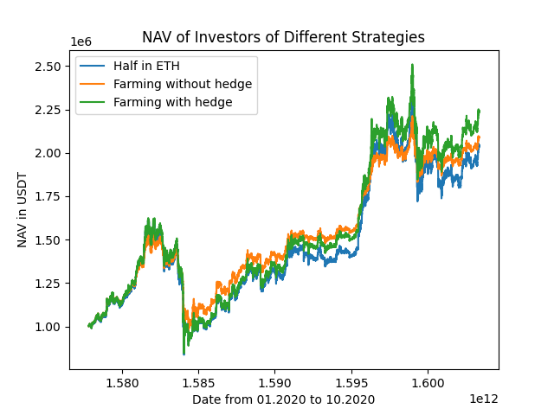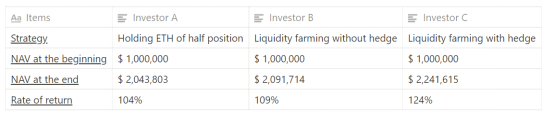During this period, the ETH price increases from \$ 128.87 to \$ 397.90, which means a 208% increase. In this case the impermanent loss is relatively small. So we can see that the performance of Investor B is slightly better than Investor A. As expected, the investor C's performance is the best again.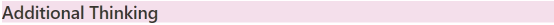1. The fundamental idea of our hedge model is effective.
2. Our model did not consider the deposit for the hedge and perpetual funding rate.
3. This model has to be improved by an optimization mechanism to reduce costs.
4. It is not really necessary to hedge in token pairs with a strong correlation between each other.
5. The hedge model can be tested separately to verify its effectiveness as an independent strategy.

By IDEG Research Team (Tao Huang, Jerry Zhu and Jack Zhang)

This content is for informational purposes only and should not be construed as investment advice.